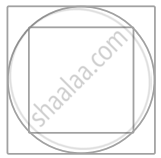# In figure, a square is inscribed in a circle of diameter d and another square is circumscribing the circle. Is the area of the outer square four times the area of - Mathematics

MCQ
True or False

In figure, a square is inscribed in a circle of diameter d and another square is circumscribing the circle. Is the area of the outer square four times the area of the inner square? Give reasons for your answer.• True

• False

#### Solution

Given diameter of circle is d.

∴ Diagonal of inner square = Diameter of circle = d

Let side of inner square EFGH be x.

∴ In right angled ΔEFG,

EG2 = EF2 + FG2   .....[By Pythagoras theorem]

⇒ d^2 = x^2 + x^2

⇒ d^2 = 2x^2

⇒ x^2 = d^2/2

∴ Area of inner square EFGH = (Side)2 = x^2 = d^2/2

But side of the outer square ABCS = Diameter of circle = d

∴ Area of outer square = d2

Hence, area of outer square is not equal to four times the area of the inner square.

Concept: Circumference of a Circle
Is there an error in this question or solution?

#### APPEARS IN

NCERT Mathematics Exemplar Class 10
Chapter 11 Area Related To Circles
Exercise 11.2 | Q 3 | Page 122

Share